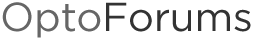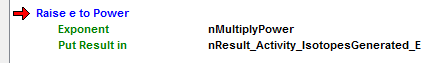# Math Formula: RaiseEToPower

Some say math is fun. I am not one of those people.

The attached document contains the handwritten math formulas I need to convert to optoscript, or charts, if that is easier. This code will need to be run every 5 seconds (the “delta t” variable) for 600 seconds.

The exponents, constants, multiplication, and divisions I believe I can do. But the one I am unable to get is the RaiseEToPower. I have highlighted in red the two formulas I am unable to code.

The good news, each formula has the correct answer next to it!

So the brave, smart, and intelligent soul that can solve this for me, I am in your debt.

Are you sure the answers are correct? Red circled formula, specifically.

@philip asks a good question here because… before you can test your code, you need to know how to tell if it worked—they call this Test-driven development (TDD) in computer science.

So, for example, if you were writing a formula (perhaps in a Subroutine!) to calculate the circumference of a circle based on its radius (e.g. 2 x PI x radius), you’d want to sanity-check a few answers.

Zero’s always a good/easy one to check first. If you plug in 0 for your radius and get something non-zero for the answer, try again.

Also, 1 is often a good test case, you’d better get something around 6.28 for this circumference example.

For the picture above, looks like for this formula circled in red, you’re trying to get a value for “d” (does this mean “population”)? Nice to use variables that are descriptive, and easier to search for, when you start coding. Also remember you get 50 characters so throwing in the units of measure (“iso” here for isotopes?) is sometimes a good idea, too. Ditto using lots of comments saying what the code is supposed to be doing.

Anyway, to calculate what I’ll call f_d_population, you apparently need values for what I’ll call: f_N_o , f_little_g, f_little_lamba, f_delta_t (what are all those?).

Note: I’m using naming convention here of a leading f_ because we’re going to use floats on the data types for all these (“why” on both of those I’ll leave for another thread).

Sometimes it helps to have other intermediate steps to see where something went wrong… I rarely get my complicated formals right on the first try!

This is true both when you’re checking your math by hand, and when you’re stepping through your code, with some known values (like we did with radius = 0 and radius = 1 on that circumference calc).

In this case, the value you’re raising e to would be one I’d create an extra variable for, perhaps called: f_e_exponent.

Perhaps also the coefficient there, (No + g) which I’ll call: f_e_coefficient
Looks like you have the one sample case (assuming the answers are correct), of:

Given: f_N_o = 319000000000.0, f_little_g = 33300000000.0, f_little_lamba = 0.00116, f_delta_t = 5
We expect: 2040000000.0 for our calculated d, based on what I’m seeing in the picture.

When I check this by hand, I get, starting with that exponent and coefficient:
f_e_exponent = -1 * f_little_lamba * f_delta_t
f_e_exponent = -1 x 0.00116 x 5 = -0.0058
f_e_coefficient = 319000000000.0 + 33300000000.0 = 352300000000.0

So e raised to that exponent = 0.9942, and when we multiply that by 352300000000.0, I get: 350256660000.0

Which is NOT the 2040000000.0 we expected, so I’m guessing that’s why @philip asked, wondering what he got….

I hesitate to hand you the proverbial fish here (the OptoScript I came up with) since that gave me roughly the same answer, but here it is anyway:

``````// initialize the example values
f_N_o =       3.19e11;
f_little_g =  3.33e10;
f_little_lamba = 0.00116;
f_delta_t = 5.0;

// do the calculations
f_e_exponent = -1 * f_little_lamba * f_delta_t;
f_e_coefficient = f_N_o + f_little_g;
f_d_pop = f_e_coefficient * RaiseEToPower(f_e_exponent);
``````

Perhaps the person that wrote all this up can help clarify what’s off in what I have above?

Also, because I can’t resist a corny joke, did anyone notice how, in her OptoScript formula, OptoMary had an f_little_lamba (whose fleece was white as snow?) Har har, so sorry, couldn’t resist there…

I get the same answer as you (~3.50e11). You get an A+.

Maybe @dman got the same answer as us and thought he was doing something wrong?

They say a picture is worth a thousand words, so here are my 2,000!

I first set the following variables:

nTarget_mCi: 10
nTimeInterval: 5
nCurrent_Target: 15

After running my attached strategy, I am able to get the “correct” answers of:

nResult_Activity: 3.189655e+011
nResult_Saturation: 3.827586e+011
nResult_IsotopesGenerated: 3.33e+010

For nMultiplyPower I get -0.0058, which may be wrong.

My answer nResult_Decayed: 1.#QNAN, which is obviously wrong. It is wrong because I am not sure how to use the RaiseEToPower() function.

I have attached the formula that I have marked up with my variable names. The attached strategy has my chart as to how I got what I got. My scientist client says the numbers are correct.

!

PS
Mary you are funny! The f_little_lamba (whose fleece was white as snow) is good!

You have the wrong part of the equation in the exponent portion of the RaiseEToPower function - for the right portion of the nResult_IsotopesGenerated equation it should be:I would recommend you think about how you are prefixing your variables - typically prefixing with ‘n’ denotes an integer, and you are working with floats. You also have some of your variables as integer when they probably should be floats as well. This could make troubleshooting difficult.

Hmmmm. I think your clients wrong. That’s a tough one.

Update: Yes, my scientist was wrong! His formula for calculating d is not the 2.04 x 10^4 as written. The answer is what we all got, the 3.50 x 10^11. That is his answer for formula N. He provided me a javascript program that better describes what we are trying to accomplish.

Thank you again Philip and Opto-Mary for the great feedback, help, and examples. I appreciate it!

Now back to more opto22 coding!